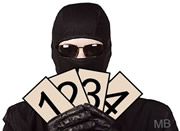Nature of Roots Practice Terms of Use    Contact Person: Donna Roberts1.
The roots of a quadratic equation may beChoose:
 rational and equal imaginary and equal irrational and equal all of the above

2.
Which equation has imaginary roots?Choose:
 x2 - 1 = 0 x2 + 2x + 1 = 0 x2 - x + 1 = 0 x2 - 9 = 0

3.
Given the equation x2 + 3x - 9 = 0. What is the product of the roots?Choose:
 9 -9 3 -3

4.
Which equation has real, rational roots that are equal roots?Choose:
 x2 - 1 = 0 x2 + 2x + 1 = 0 x2 - x + 1 = 0 x2 + x + 1 = 0

5.
What is the sum of the roots of the equation
2x2 - 3x + 9 = 0?Choose:
 3/2 2 2/3 1/2

6.
The roots of the equation x2 - 3x - 2 = 0Choose:
 are real, rational, and equal. are real, rational, and unequal. are real, irrational, and unequal. are imaginary.

7.
Which of the following could not be the roots of a quadratic equation?Choose:
 {4,5} {3} {2i, -2i} {3 + 4i, -3 - 4i}

8.
Which quadratic equation has roots 6i and -6i ?Choose:
 x2 - 36 = 0 x2 + 12x + 36 = 0 x2 + 36 = 0 x2 - 12x - 36 = 0

9.
If one of the roots of the equation
x2 - 6x + k = 0 is 4, what is the other root?Choose:
 6 2 3 -10

10.
Which equation has imaginary roots?Choose:
 x(5 + x) = 8 x(5 - x) = -3 x(x + 6) = -10 (2x + 1)(x - 3) = 7